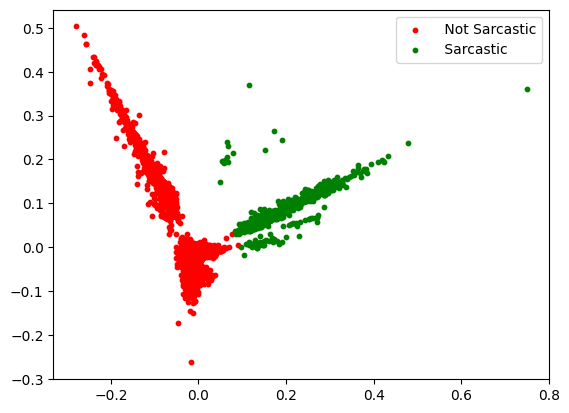# Clustering Text Documents using K-Means in Scikit Learn

Clustering text documents is a typical issue in natural language processing (NLP). Based on their content, related documents are to be grouped. The k-means clustering technique is a well-liked solution to this issue. In this article, we’ll demonstrate how to cluster text documents using k-means using Scikit Learn.

### K-means clustering algorithm

The k-means algorithm is a well-liked unsupervised learning algorithm that organizes data points into groups based on similarities. The algorithm operates by iteratively assigning each data point to its nearest cluster centroid and then recalculating the centroids based on the newly formed clusters.

### Preprocessing

Preprocessing describes the procedures used to get data ready for machine learning or analysis. It frequently involves transforming, reformatting, and cleaning raw data and vectorization into a format appropriate for additional analysis or modeling.

#### Steps

2. Preprocessing of text in case the text is loaded instead of manually adding it to the code
3. Vectorizing the text using TfidfVectorizer
4. Reduce the dimension using PCA
5. Clustering the documents
6. Plot the cluster using matplotlib

## Python3

 `# import the necessary libraries ` `import` `json ` `import` `numpy as np ` `import` `pandas as pd ` `from` `sklearn.feature_extraction.text ``import` `TfidfVectorizer ` `from` `sklearn.decomposition ``import` `PCA ` `from` `sklearn.cluster ``import` `KMeans ` `import` `matplotlib.pyplot as plt ` ` `  `# Dataset link:  ` `# https://github.com/PawanKrGunjan/Natural-Language-Processing/blob/main/Sarcasm%20Detection/sarcasm.json ` `df``=``pd.read_json(``'sarcasm.json'``) ` ` `  `# Extract the sentence only ` `sentence ``=` `df.headline ` ` `  `# create vectorizer ` `vectorizer ``=` `TfidfVectorizer(stop_words``=``'english'``) ` ` `  `# vectorizer the text documents ` `vectorized_documents ``=` `vectorizer.fit_transform(sentence) ` ` `  `# reduce the dimensionality of the data using PCA ` `pca ``=` `PCA(n_components``=``2``) ` `reduced_data ``=` `pca.fit_transform(vectorized_documents.toarray()) ` ` `  ` `  `# cluster the documents using k-means ` `num_clusters ``=` `2` `kmeans ``=` `KMeans(n_clusters``=``num_clusters, n_init``=``5``, ` `                ``max_iter``=``500``, random_state``=``42``) ` `kmeans.fit(vectorized_documents) ` ` `  ` `  `# create a dataframe to store the results ` `results ``=` `pd.DataFrame() ` `results[``'document'``] ``=` `sentence ` `results[``'cluster'``] ``=` `kmeans.labels_ ` ` `  `# print the results ` `print``(results.sample(``5``)) ` ` `  `# plot the results ` `colors ``=` `[``'red'``, ``'green'``] ` `cluster ``=` `[``'Not Sarcastic'``,``'Sarcastic'``] ` `for` `i ``in` `range``(num_clusters): ` `    ``plt.scatter(reduced_data[kmeans.labels_ ``=``=` `i, ``0``], ` `                ``reduced_data[kmeans.labels_ ``=``=` `i, ``1``],  ` `                ``s``=``10``, color``=``colors[i],  ` `                ``label``=``f``' {cluster[i]}'``) ` `plt.legend() ` `plt.show()`

Output:

```                                                document  cluster
16263  study finds majority of u.s. currency has touc...        0
5318   an open and personal email to hillary clinton ...        0
12994        it's not just a muslim ban, it's much worse        0
5395   princeton students confront university preside...        0
24591     why getting married may help people drink less        0```Text clustering using KMeans

Whether you're preparing for your first job interview or aiming to upskill in this ever-evolving tech landscape, GeeksforGeeks Courses are your key to success. We provide top-quality content at affordable prices, all geared towards accelerating your growth in a time-bound manner. Join the millions we've already empowered, and we're here to do the same for you. Don't miss out - check it out now!

Previous
Next